Courses

# Test: Reaction Mechanism- 3

## 20 Questions MCQ Test Organic Chemistry | Test: Reaction Mechanism- 3

Description
This mock test of Test: Reaction Mechanism- 3 for Chemistry helps you for every Chemistry entrance exam. This contains 20 Multiple Choice Questions for Chemistry Test: Reaction Mechanism- 3 (mcq) to study with solutions a complete question bank. The solved questions answers in this Test: Reaction Mechanism- 3 quiz give you a good mix of easy questions and tough questions. Chemistry students definitely take this Test: Reaction Mechanism- 3 exercise for a better result in the exam. You can find other Test: Reaction Mechanism- 3 extra questions, long questions & short questions for Chemistry on EduRev as well by searching above.
QUESTION: 1

Solution:
QUESTION: 2

Solution:
QUESTION: 3

### The products formed in the following reaction is: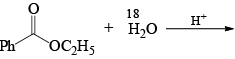Solution:
QUESTION: 4

In the reaction sequence,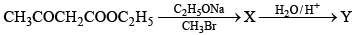The product (Y) is:

Solution:
QUESTION: 5

The major product of the reaction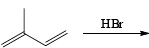Solution:
QUESTION: 6

The major product formed in the following reaction.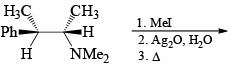Solution:
QUESTION: 7

The major product formed in the following reaction.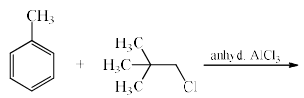Solution:
QUESTION: 8

The major product obtained in the following reactionSolution:
QUESTION: 9

The major product formed in the following reaction is: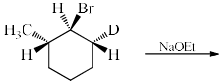Solution:

NaOEt is a strong base and it will abstract the acidic proton from the alpha carbon. Dueterium is abstracted from alpha carbon because it is show more +I effect than hydrogen. After that then elimination will occur with bromide as leaving group.

Hence C

QUESTION: 10

Choose the correct order of reactivity for dehydration of the given alcoho ls using concentrated sulfuric acid.

Solution:
QUESTION: 11

Electrophilic nitrations of the following compounds follow the trend: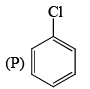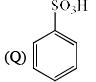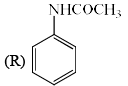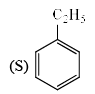Solution:
QUESTION: 12

The set of products formed in the following reaction is: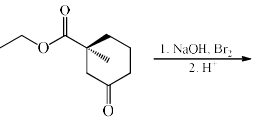Solution:
QUESTION: 13

What would be the final major product of the following chemical reaction if it is carried out twice, one at 5 Co and the second time at 45 C0?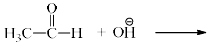Solution:
QUESTION: 14

What is the major product if HBr (in excess) is added to H2C = CH – CH2 – OH

Solution:
QUESTION: 15

The reaction of sodium ethoxide with ethyl iodide to form diethyl ether is termed

Solution:
QUESTION: 16

What is the final product after the following reaction has gone to completion?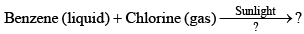Solution:
QUESTION: 17

The major product formed in the following reaction is: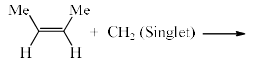Solution:
QUESTION: 18

Among the bro mides I-III given below, the order of their reactivity in the SN1 reaction is: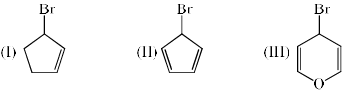Solution:

SN1 reaction proceeds through carbocation as intermediate. Br- removed and made up carbocation in option A, B, C then we check the stability of carbonations. C is more stable because carbocation of C is aromatic, A is non aromatic and B is anti aromatic. So order of stability is:

Aromatic > Non-Aromatic > Anti Aromatic. Then more stable carbocation higher will be the reactivity and faster will be the SN1 reaction.

Hence C is correct.

QUESTION: 19

The major product formed in the reaction benzoic acid with isobutylene in the presence of a catalytic amount of sulfuric acid is:

Solution:
QUESTION: 20

Among the following compounds, the one that undergoes deprotonation most readily in the presence of a base, to form a carbanion is:

Solution: